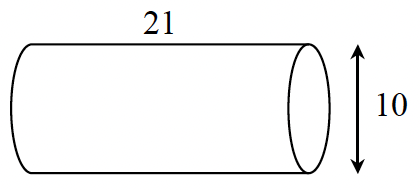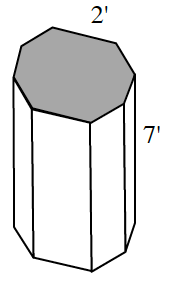### Home > GC > Chapter 9 > Lesson 9.2.4 > Problem9-89

9-89.

Find the volumes of the solids below.

1. cylinderRecall the formula for the volume of a cylinder.

$\text{Cylindrical volume}=(\text{area of the base})\times(\text{height})$

1. regular octagonal prismDivide the octagonal base into congruent triangles to find its area.

Now use the formula $(\text{volume})=(\text{area of the base})\times(\text{height})$ to find the volume of the prism.

$\text{Base Area}\approx19.3\text{ units}^2$
$\text{Volume}\approx(19.3)(7)\approx135.2\text{ units}^3$